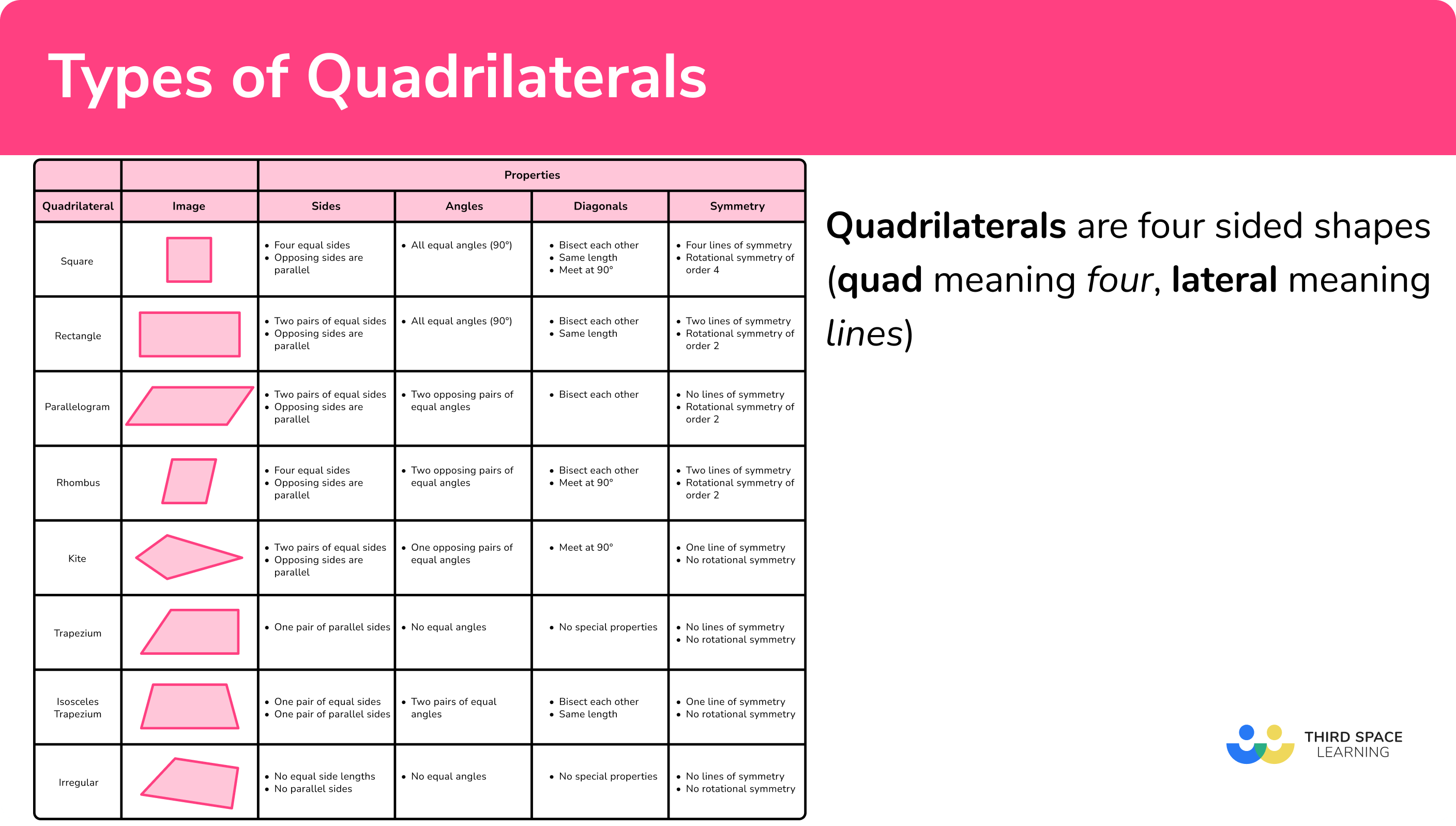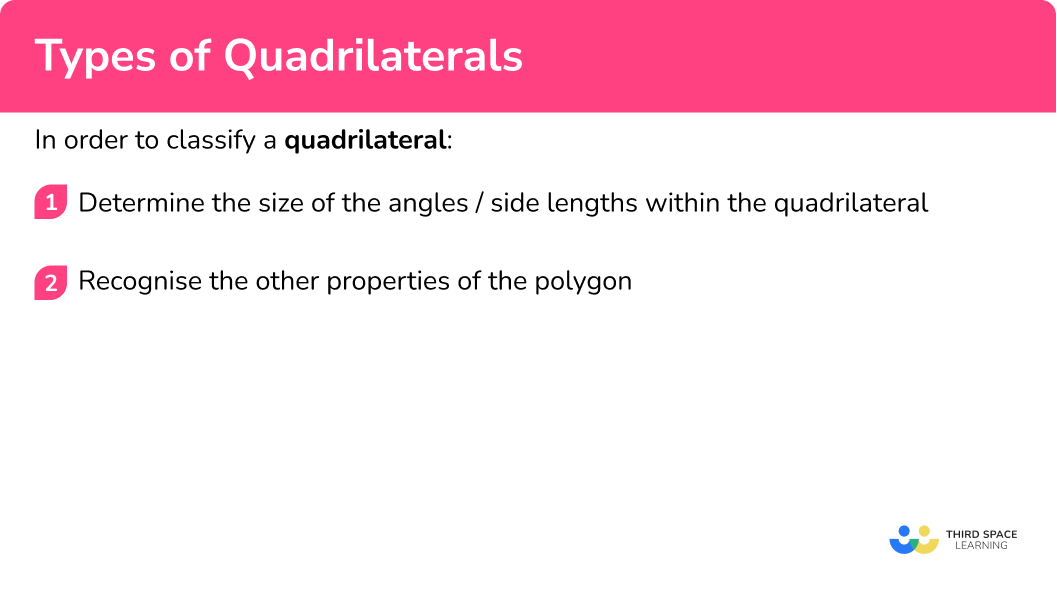Here we will learn about types of quadrilaterals, including their names, properties and applications.

There are also types of quadrilaterals worksheets based on Edexcel, AQA and OCR exam questions, along with further guidance on where to go next if you’re still stuck.

## What are types of quadrilaterals?

For example,

• The sum of interior angles of a quadrilateral total 360^{\circ} .
• Quadrilaterals can be convex or concave.
A convex polygon is a polygon with all the interior angles less than 180^{\circ} .
A concave polygon has at least one angle that is greater than 180^{\circ} (or a reflex angle).
The minimum number of edges of a polygon for it to be able to be concave is four (specifically an arrowhead).

The arrowhead has a few special properties. The main property that makes it different to other quadrilaterals is that the diagonals bisect externally to the polygon and are perpendicular to one another. Similar to a kite, the arrowhead has two pairs of adjacent sides that are equal length.

### What are types of quadrilaterals?## How to classify a quadrilateral

In order to classify a quadrilateral:

1. Determine the size of the angles/side lengths within the quadrilateral.
2. Recognise the other properties of the polygon.

### How to classify a quadrilateral### Related lessons on polygons

Types of quadrilaterals is part of our series of lessons to support revision on polygons. You may find it helpful to start with the main polygons lesson for a summary of what to expect, or use the step by step guides below for further detail on individual topics. Other lessons in this series include:

### Example 1: square or rectangle

1. Determine the size of the angles/side lengths within the quadrilateral.

As the sides are perpendicular, we can say that this quadrilateral is either a square or a rectangle. We need to determine the height of the quadrilateral as if the height is the same as the width, it is a square, If not, it is a rectangle.

We need to use Pythagoras’ theorem as the triangle used contains a right angle and we do not know the other angles in the triangle.

x^{2}=20^{2}-12^{2}

x^{2}=400-144

x^{2}=256

x=16

2Recognise the other properties of the polygon.

As the height is not equal to the width of the quadrilateral and all the angles are equal to 90^{\circ} , the quadrilateral is a rectangle.

### Example 2: parallelogram or rhombus

Determine what type of quadrilateral shape ABCD is.

As angles in a triangle total 180^{\circ} , angle ABD = 78^{\circ} . As the opposite sides are parallel, co-interior angles within the parallel lines total 180^{\circ} .

• Angle ADC = 180 - (32 + 70) = 78^{\circ}
• Angle ABC = 180 - 32 = 148^{\circ}
• Angle BCD = 180 - 148 = 32^{\circ}

We have two pairs of equal angles at opposing vertices ( 148^{\circ} and 32^{\circ} ). To determine whether the quadrilateral is a rhombus, the diagonals must bisect at 90^{\circ} . This means that the two triangles ABD and BCD must be symmetrical about the diagonal BD .

Angle BDC = 148 - 70 = 78^{\circ} . As these are not the same, the quadrilateral ABCD is a parallelogram.

### Example 3: quadrilateral with symmetry

The polygon below is a quadrilateral. The points M and N are midpoints of the lines AB and CD respectively. The quadrilateral has one line of symmetry along MN . Angle AMN = 90^{\circ} . By using angle properties, determine the type of quadrilateral.

As the line MN is a line of symmetry, the angle ABC = 43^{\circ} and angle ADC = 137^{\circ} .

Adding angles ABC and BCD together totals 180^{\circ} so the lines AB and CD are parallel. The lines BC and AD must be the same length as they are symmetrical about the line MN .

The quadrilateral is an isosceles trapezium.

### Example 4: multiple triangles

Three different triangles are placed together to form a quadrilateral ABCD . Determine the classification of the quadrilateral.

Angle DAE = 180 - (90+75) = 15^{\circ} as angles in a triangle total 180^{\circ} .

As AB and CD are parallel, angle BAE = 75^{\circ} (alternate angles in parallel lines are equal).

As ABE is an isosceles triangle, angle ABE = 75^{\circ} and so angle AEB = 180 - (75+75) = 30^{\circ} .

As angles on a straight line total 180^{\circ} , angle BEC = 180 - (75 + 30) = 75^{\circ} .

This finally allows us to calculate angle CBE = 180 - (55+75) = 50^{\circ} .

We now have

The four interior angles of the quadrilateral are: 90^{\circ}, 55^{\circ}, 90^{\circ} and 125^{\circ} .

This confirms that the quadrilateral is trapezium as it only has one pair of parallel sides and no lines of symmetry.

### Example 5: two trapezia

Two congruent trapezia are joined together to form another quadrilateral. Determine the classification of the polygon ABCD .

As the lines AB and CD are parallel, EAC = 180 - 52 = 128^{\circ} (co-interior angles total 180^{\circ} ). Also, for the same reason, angle AEF = 180 - 64 = 116^{\circ} . As the two trapezia are congruent, we can fill in the rest of the angles on the diagram.

Looking at the side lengths of the quadrilateral, we can say that as AE = 3cm, CF = 3cm so AB = CD = 8cm .

All the side lengths are equal, with two pairs of parallel sides ( AD and BC are also parallel) and so the quadrilateral is a rhombus.

### Example 6: interior and exterior angles

Use the information in the diagram to determine what type of quadrilateral ABCD is.

As angles on a straight line total 180^{\circ} , we can calculate the angles ADC and ABC.

ADC = 180 - 88 = 92^{\circ}

ABC = 180 - 84 = 96^{\circ}

The remaining interior angle at C is therefore equal to 360 - (92 + 96 + 85) = 87^{\circ} .

The four interior angles of the quadrilateral are 85^{\circ}, 92^{\circ}, 96^{\circ}, and 87^{\circ} . As all of the angles are different sizes, the polygon is an irregular quadrilateral.

### Common misconceptions

• Angles in polygons

Make sure you know your angle properties. Getting these confused causes quite a few misconceptions.

• Angles in a triangle total 180^{\circ} .
• Angles in a quadrilateral total 360^{\circ} .
• Angles on a straight line total 180^{\circ} .

There are many quadrilaterals and it is common to confuse the properties, especially for a rhombus, parallelogram, or trapezium.

As well as this, stating that the polygon is a quadrilateral is not enough information for a classification.

### Practice types of quadrilaterals questions

1. Correctly classify the following quadrilateral.ParallelogramRectangleRhombusSquareUsing Pythagoras’ theorem, we can calculate the height of the quadrilateral.

x^2=(8\sqrt{2})^2-8^2\\ x^2=128-64\\ x^2=64\\ x=8cm

The height and the width of the polygon are equal and all angles are 90^{\circ} .

2. Determine what type of quadrilateral shape ABCD is.RhombusParallelogramTrapeziumDiamondAngles in a triangle total 180^{\circ} so angle COD = 180-(30 + 64) = 86^{\circ} . As angle COD is not a right angle and the opposite sides of the shape are parallel and also not right angles, shape ABCD is a parallelogram.

3. ACE is a triangle. The line BD is parallel to line
AE with angle BDE = 140^{\circ} and angle CBD = 40^{\circ} . Classify the polygon ABDE .Isosceles triangleEquilateral triangleIsosceles trapeziumParallelogramAngle BAE = 40^{\circ} (corresponding angles are equal).
Angles CDB = 180 – 140 = 40^{\circ} (angles on a straight line total 180^{\circ} ).
Angle BAE = CBD = 40^{\circ} (corresponding angles are equal).
Angle DEA = 180 – 140 = 40^{\circ} (co-interior angles total 180^{\circ} ).

The quadrilateral has one pair of parallel lines with two pairs of equal angles meaning that the quadrilateral has 1 line of symmetry.

4. Three congruent isosceles triangles are joined together in the shape below. E is the midpoint of the line AB . Classify the polygon ABCD .Isosceles trapeziumTrapeziumParallelogramIsosceles triangleUsing angles in isosceles triangles we haveAB and CD are parallel because their co-interior angles total 180^{\circ} . As the angles BCA and BAD are not equal, the polygon does not have a line of symmetry and so the polygon ABCD is a trapezium.

5. A trapezium is duplicated and rotated 180^{\circ} . Side A’D’ is joined to side AD . What is the classification of the new polygon created?HexagonSquareRectangleParallelogramAs the two lines in the middle of the diagram join, the only interior angles remaining are all 90^{\circ} as BC is perpendicular to AB and CD . The quadrilateral  C’BCB’ is a rectangle.

6. Use the angles provided in the diagram to classify the quadrilateral ABCD .ParallelogramRhombusTrapeziumRectangleAlternate angles in parallel lines are equal so angle BAC = angle ACD = 31^{\circ} .
Angle AOB = 180-(31+53) = 96^{\circ} as angles in a triangle total 180^{\circ} . As the angle AOB is not a right angle, and there are two pairs of parallel sides, ABCD is a parallelogram.

### Types of quadrilaterals GCSE questions

1. (a) Plot one coordinate to make an isosceles trapezium using the other three points drawn below.(b) Using the same set of axes, plot the coordinate of the point to make a parallelogram.

(2 marks)

(a)
(-2,4)

(1)

(b)
(6,4)

(1)

2. Below are the names of five quadrilaterals.

(a) Which polygon has only one line of symmetry?

(b) Which polygon has a rotational symmetry of 4 ?

(c) Which polygon has only one pair of parallel lines?

(3 marks)

(a)
Kite

(1)

(b)
Square

(1)

(c)
Trapezium

(1)

3. (a) Below is a circle with two tangents meeting at point A . Given that a tangent is perpendicular to the radius at the same point, and AB = AD , show that the angle BCD = 132^{\circ} . Give reasons for your answer.(11 marks)

(a)
Angle BAD = 180 – 132 = 48^{\circ} .

(1)

Angles on a straight line total 180^{\circ} .

(1)

Angle ABC = ADC = 90^{\circ} .

(1)

The tangent meets the radius at 90^{\circ} .

(1)

BCD = 360-(90+90+48) .

(1)

Angles in a quadrilateral total 360^{\circ} .

(1)

Description and Angle BCD = 132^{\circ} .

(1)

(b)
Kite

(1)

Two pairs of non-parallel sides.

(1)

Two pairs of sides of equal length.

(1)

One pair of opposite angles are equal.

(1)

## Learning checklist

You have now learned how to:

• Plot specified points and draw sides to complete a given polygon
• Distinguish between regular and irregular polygons based on reasoning about equal sides and angles
• Compare and classify geometric shapes based on their properties and sizes and find unknown angles in any triangles, quadrilaterals, and regular polygons
• Describe, sketch and draw using conventional terms and notations: points, lines, parallel lines, perpendicular lines, right angles, regular polygons, and other polygons that are reflectively and rotationally symmetric
• Derive and use the sum of angles in a triangle and use it to deduce the angle sum in any polygon, and to derive properties of regular polygons

## Still stuck?

Prepare your KS4 students for maths GCSEs success with Third Space Learning. Weekly online one to one GCSE maths revision lessons delivered by expert maths tutors.

Find out more about our GCSE maths tuition programme.